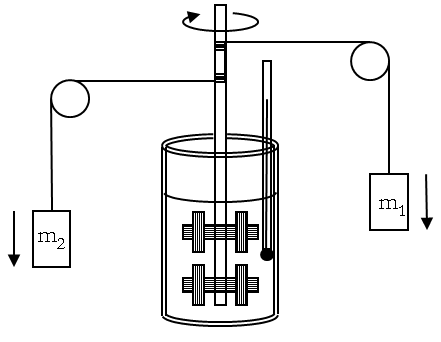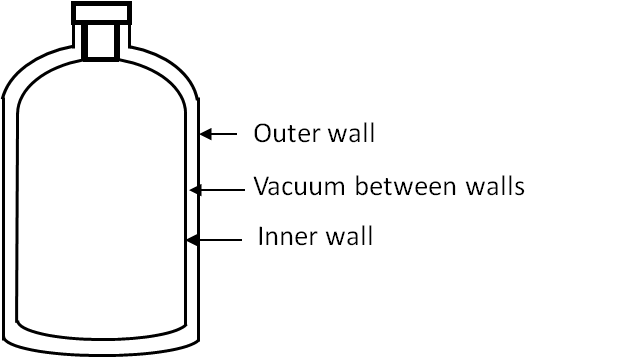## Section19.2Nature of Heat

Prior to the nineteenth century, scientists thought that the sense of hot and cold was related with the amount of heat a body contained. The theory was called the caloric theory. In the caloric theory, heat is considered to be a “weightless fluid” that flowed from a hot body to a cold body with the total quantity being conserved. This viewpoint of heat is no longer held since experiments have suggested better ways of looking at the phenomena involving heat as we will see below.

In 1798 Count Rumford, born as Benjamin Thompson in Massachusetts, USA, presented a paper to the Royal Society of Great Britain in which he described the results of a series of experiments conducted in Munich, Germany concerning heat associated with the boring of cannons. He had observed that in the drilling of canons, the cannons became blazingly hot and water poured to cool them also became very hot. After a series of careful measurements he found that the amount of heat released into water would be enough to completely melt the canon if it could be returned to the metal. Therefore, he concluded that more heat was being released in the drilling process than could have been originally contained in the metal. This observation contradicted the caloric theory. Rumford concluded that it was the mechanical process of boring that was responsible for heat production and not the supposedly heat present in the metal beforehand. In his own words,

“It appears to me extremely difficult, if not impossible, to form any distinct idea of anything capable of being excited and communicated in the manner that heat was excited and communicated in these experiments, except it be motion.”

As a result, physicists consider heat as a form of energy in transit as opposed to an energy such as kinetic energy that a system contains. When a body at higher temperature comes in a direct contact with another body at a lower temperature, then, the energy transferred from the body at higher temperature to the body at lower temperature is said to be heat.

Rumford also calculated the amount of heat produced in the boring of a cannon and related it to the mechanical energy put in for boring thereby finding the first reported value of the mechanical equivalent of heat. His value expressed in the unit of heat (calories) and the British unit of mechanical energy (Foot-pound) would be $3.15$ foot-pound per calorie, which is approximately $1.5\%$ higher than the presently accepted value. Count Rumford also invented many other useful devices, such as the kitchen range, the double boiler, the pressure-cooker and the drip coffee maker.

A clearer demonstration of the mechanical equivalence of heat was provided by James Joule in England in 1840's. To demonstrate the equivalence of mechanical energy and heat, Joule constructed a device where he could measure mechanical energy and thermal energy at the same time. In his device, a sketch of which is shown in Figure 19.2.1, paddle wheels attached to a shaft were placed in a set amount of water in a copper cylinder. The shaft rotated when the strings wrapped over it unwound when the two masses fell. Joule expected that the rotating paddle wheels would force the water molecules to rub against one another and produce heat as a result of the friction between the paddle wheel and the liquid.Figure 19.2.1. Joule's experiment. As the masses fall, the rotating paddle wheel forces the water molecules to rub against each other and produce heat. Heat measured in calories from the rise in temperature and work measured in Joules give the mechanical equivalent of heat as $1\text{ cal} = 4.1855\text{ J}\text{.}$

The loss in potential energy of the falling masses provided a measure of the mechanical energy introduced in the system and the change in temperature was measured to figure out the amount of thermal energy. In Joule's set-up, the change in temperature was expected to be very small, approximately 0.2 degrees Celsius. To measure such small differences in temperature Joule constructed his own thermometer, and conducted experiments in a wine cellar, which provided a stable temperature environment. He found that the loss in the mechanical energy was directly proportional to the rise in the temperature. He was also able to find a numerical value of the proportionality constant between work and thermal energy, which we now call mechanical equivalence of heat.

### Subsection19.2.1Unit of Heat

The common unit of heat, called $\text{calorie (cal)}\text{,}$ is defined as the amount of heat needed to raise the temperature of $1\text{ g}$ of water by $1^\circ\text{C}$ from $14.5^{\circ}\text{C}$ to 1$15.5^{\circ}\text{C}$ at $1\text{ atm}$ of pressure, while the SI unit of the mechanical work is $1\text{ Joule (J)}\text{.}$ The proportionality constant between the work and the thermal energy is presently known to be $4.1855\text{ J/cal}\text{.}$

\begin{equation} 1\text{ calorie} = 4.1855\text{ Joules}.\ \ \ \text{(mechanical equivalence)}\tag{19.2.1} \end{equation}

In engineering practice in the United States and the Great Britain, heat is also expressed in a unit called the British Thermal Unit or $\text{BTU}\text{.}$ One $\text{BTU}$ is the amount of heat needed to raise the temperature of one pound of water by one degree Fahrenheit. It is equal to $252\text{ calories}\text{.}$

\begin{equation} 1\text{ BTU} = 252\text{ calories}.\ \ \ \text{(units of heat)}\tag{19.2.2} \end{equation}

Note that nutrition professionals also use a unit of energy called $\text{Calorie}\text{,}$ written with capital letter C, which is not the same as $1\text{ calorie}\text{,}$ written with a small letter c as defined above, but instead $1000\text{ calories}\text{.}$

\begin{equation} 1\text{ Nutrition Calorie} = 1000\text{ calories}.\tag{19.2.3} \end{equation}

### Subsection19.2.2Thermal Contact

If heat can flow between two bodies, then we say that they are in thermal contact with one another and their contact is often called a diathermal wall. If two bodies are insulated from each other so that no heat can be exchanged between them, then the wall is called an adiabatic wall.

In a Dewar flask a vacuum between the outer wall and the inner wall serves as an adiabatic wall and prevents the flow of heat.Since energy from a hot body to a cold body can go through vacuum by the mechanism of radiation, in principle, no adiabatic wall can insulate 100%. However, as a practical matter, we can block the heat transfer to a desired degree in most situations of interest.

### Subsection19.2.3Microscopic Picture of Heat Flow

Microscopically, heat is related with the change in the translation, vibration and rotation of atoms and molecules in a substance. When we touch a hot cup, the vibrational energy of the molecules of the cup are transferred to the molecules of the skin by physical impulse thereby increasing their energy, which gives us the sensation of heat. This mechanism where the energy of a body is changed without moving its boundaries macroscopically is an example of heat transfer, and the amount of energy transferred in this way is what we have been calling heat.

In a Joule experiment $1\text{ kg}$ of liquid water in a $200\text{ g}$ copper container is stirred by flywheels when two blocks each weighing $5\text{ kg}$ drop by a height of $1.5\text{ m}\text{.}$ Assuming that all heat generated by the resistance against the motion of the flywheel goes in raising the temperature of the water and the container, what will be the rise in the temperature? Assume that kinetic energy of blocks do not increase much during the fall.

Hint

Use energy flow balance.

$0.035^{\circ}\text{C}\text{.}$

Solution

The potential energy of the two blocks is converted to the kinetic energy of the flywheels and kinetic energy of the blocks. We ignore KE of blocks and assume the KE of flywheels is expended in raising the temperature of the water, the container and the flywheels. We will ignore the energy in raising the temperature of the container and the flywheels since they are very small compared to the energy in raising the temperature water. Energy to raise the temperature of wateris given by the following formula

\begin{equation*} m_{w}\, c_{w} \, |\Delta T|, \end{equation*}

where $m_{w}$ is mass of water, $c_{w}$ the specific heat of water with value $1.0\text{ cal/g.}^\circ\text{C}\text{,}$ and $|\Delta T|$ the change in its temperature. We equate this to the energy lost by the falling blocks to get

\begin{equation*} m_{w}\, c_{w} \, |\Delta T| \approx 2\times m g h. \end{equation*}

Solving this for $|\Delta T|\text{,}$ we get

\begin{align*} |\Delta T| \amp = \frac{2 m g h}{m_{w}\ c_{w}} \\ \amp = \frac{2\times 5\ \text{kg} \times 9.81\ \text{m/s}^2 \times 1.5\ \text{m}}{1000\ \text{g}\times 1.0\ \text{cal/g}.^{\circ}\text{C}\times 4.186\ \text{J/cal}} \\ \amp \approx 0.035^{\circ}\text{C}. \end{align*}

A $30\text{-hp}$ motor is to rotate the paddles in the Joule mechanical equivalent of heat experiment. Assuming the electrical energy supplied to the motor ends up heating $3\text{ kg}$ of water initially at $20^{\circ}\text{C}\text{.}$ How long the motor must run for the temperature to rise to $30^{\circ}\text{C}\text{.}$

Hint

Use energy conservation.

$5.7\text{ sec}\text{.}$

Solution

The unit horse power ($\text{hp}$) is a unit of power and has the following conversion to Watts ($\text{W}$).

\begin{equation*} 1\text{ hp} = 730\text{ W}. \end{equation*}

Therefore, the power of the motor is

\begin{equation*} P = 30\times 730\text{ W} = 21,900\text{ W}. \end{equation*}

The energy supplied by motor in time $\Delta t$ will be $P\Delta t\text{.}$ This energy goes towards heating water. The energy needed to raise the temperature of a mass $m$ of water by temperature $\Delta T$ is $mc\Delta T\text{.}$ The energy conservation gives

\begin{equation*} P\Delta t = mc\Delta T. \end{equation*}

Therefore,

\begin{align*} \Delta t \amp = \frac{mc\Delta T}{P}\\ \amp = \frac{3 \text{ kg}\times 4,186\text{ J/kg}.^{\circ}\text{C}\times 10^{\circ}\text{C}}{21,900\text{ W}}\\ \amp = 5.7\text{ sec}. \end{align*}

A heating element has a resistance ($R$) of 2 Ohms($\Omega$). When a current $I$ passes through a conductor of resistance $R\text{,}$ the conductor uses energy at a rate of $I^2R\text{.}$ The heating element is placed in $500\ \text{cm}^{3}$ of liquid water at $20^{\circ}\text{C}$ in a Dewar flask, and a current of $2.5\text{ amperes}$ is passed for 30 sec. Find the final temperature of water. (Units: $1\ \text{A}^2.\Omega = 1\ \text{W}\text{.}$)

Hint

Use energy balance.

$20.2^\circ\text{C}$

Solution

The electrical energy per unit time dissipated in the heating element is

\begin{equation*} P = I^2 R = (2.5\ \text{A})^2\times 2\ \Omega = 12.5\ \text{W}. \end{equation*}

The energy goes towards changing the temperature of the element and the water. We ignore the energy going into heating the element since it is much smaller compared to the energy to heat the water. In time $\Delta t$

\begin{equation*} m_{w}c_\text{w}\Delta T \approx P\Delta t \ \ \Longrightarrow\ \ \Delta T = \frac{P\Delta t}{m_{w}c_\text{w}}. \end{equation*}

Here $\Delta T = T_f - T_i\text{,}$ where $T_i$ and $T_f$ are the initial and final temperatures respectively. Putting in the numbers, noting that $500\text{ cc}$ of water has mass $0.500\text{ kg}\text{,}$ we get

\begin{align*} T_f \amp = 20^{\circ}\text{C} + \frac{12.5\ \text{W}\times 30\ \text{s}}{ 0.5\ \text{kg}\times 4,186\ \text{J/kg}.^{\circ}\text{C}}\\ \amp = 20.2^{\circ}\text{C}. \end{align*}